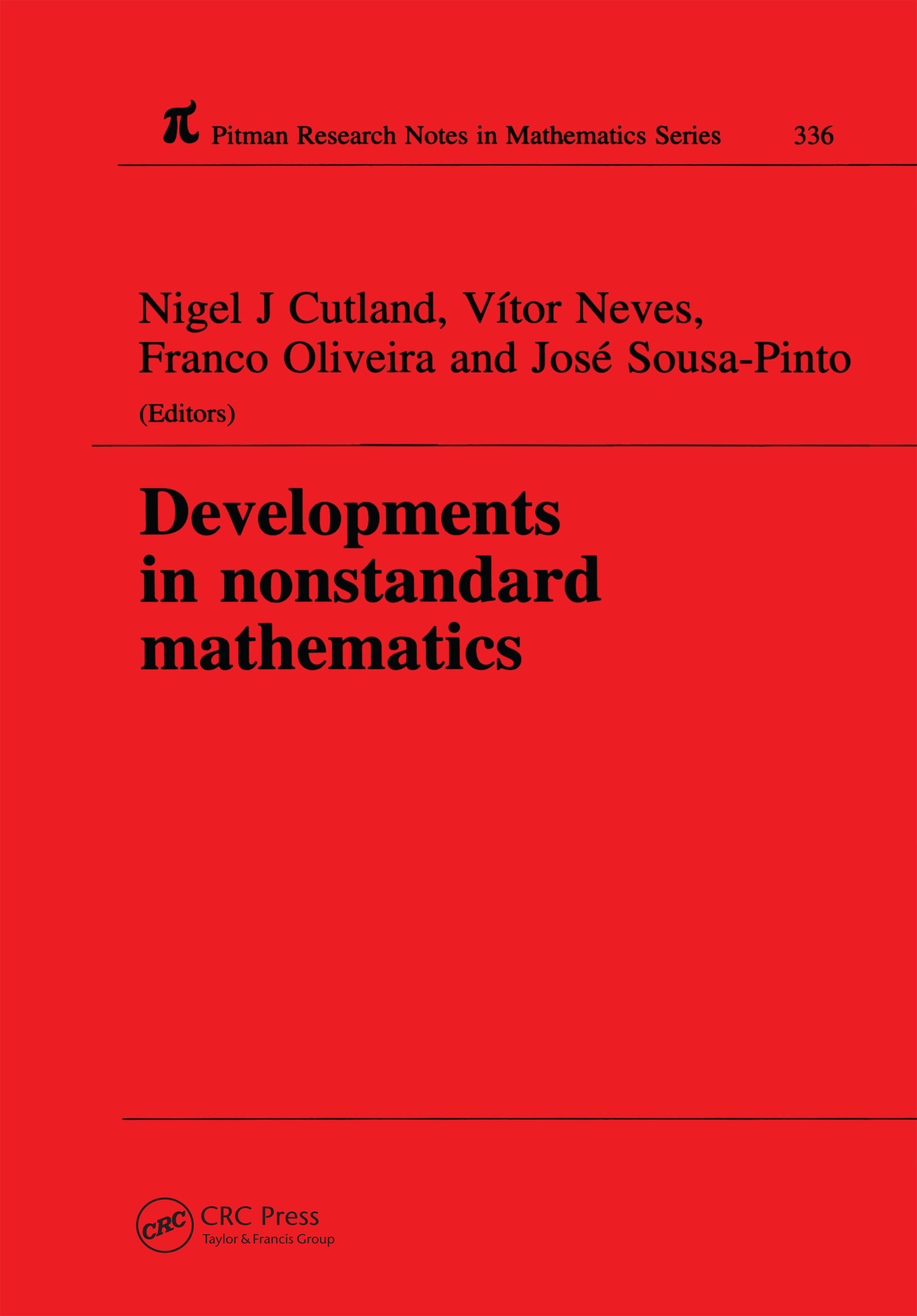Developments in Nonstandard Mathematics

1st Edition

Chapman and Hall/CRC

272 pages

Purchasing Options:\$ = USD
Hardback: 9780582279704
pub: 1995-11-01
SAVE ~\$37.00
Currently out of stock
\$185.00
\$148.00
x

FREE Standard Shipping!

Description

This book contains expository papers and articles reporting on recent research by leading world experts in nonstandard mathematics, arising from the International Colloquium on Nonstandard Mathematics held at the University of Aveiro, Portugal in July 1994. Nonstandard mathematics originated with Abraham Robinson, and the body of ideas that have developed from this theory of nonstandard analysis now vastly extends Robinson's work with infinitesimals. The range of applications includes measure and probability theory, stochastic analysis, differential equations, generalised functions, mathematical physics and differential geometry, moreover, the theory has implicaitons for the teaching of calculus and analysis.

This volume contains papers touching on all of the abovbe topics, as well as a biographical note about Abraham Robinson based on the opening address given by W.A>J> Luxemburg - who knew Robinson - to the Aveiro conference which marked the 20th anniversary of Robinson's death. This book will be of particular interest to students and researchers in nonstandard analysis, measure theory, generalised functions and mathematical physics.

The infinitesimal rule of three

Nonstandard methods in the precalculus curruculum

Difference quotients and smoothness

Continuous maps with special properties

Some nonstandard methods in geometric topology

Delayed bifurcations in perturbed systems analysis of slow passage of Suhl-threshold

Functional analysis and NSA

Near-standard compact internal linear operators

Discrete Fredholm's equations

Nonstandard theory of generalized functions

Representing distributions by nonstandard polynomials

Contributions of nonstandard analysis to partial differential equations

Loeb measure theory

Unions of Loeb nullsets: the context

Gredient lines and distributions of functionals in infinite dimensional Euclidean spaces

Nonstandard flat integral representation of the free Euclidean field and a large deviation bound for the exponential interaction

Nonstandard analysis in selective unierses

A neometric survey

Long sequences and neocompact sets